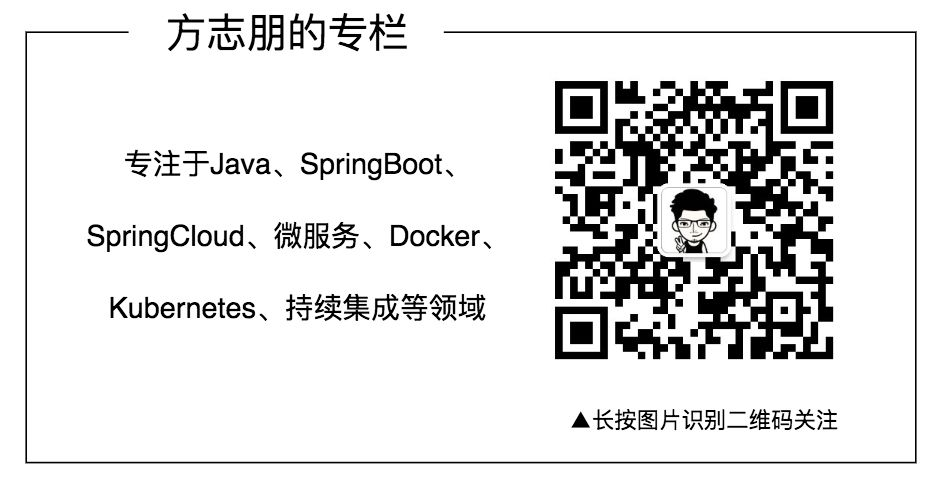# 美团面试题：String s = new String("111")会创建几个对象？

04/18 17:20

## String不可变吗？

public class App {    public static void main(String[] args) {        String a = "111";        a = "222";        System.out.println(a);    }}

222

Stirng a="111"；

User user = new User()

String 为什么是不可变的？

public final class String implements java.io.Serializable, Comparable<String>, CharSequence {    private final char value[];    public String() {        this.value = "".value;    }    public String(String original) {        this.value = original.value;        this.hash = original.hash;    }    public String(char value[]) {        this.value = Arrays.copyOf(value, value.length);    }}   

String 类是final修饰

String存储内容使用的是char数组

char数组是final修饰

## 这里就得复习一下，final有啥用？

/** * Description: final修饰变量 * @author : 田维常 * 欢迎关注：java后端技术全栈 */public class FinalDemo {    private final String name;    public FinalDemo(String name) {        this.name = name;    }    public FinalDemo() {    }}

/** * Description: * * @author : 田维常 * @date : 2020/11/3 * 欢迎关注公众号：java后端技术全栈 */public class StringDemo {    public static void main(String[] args) {        String name = "老田";        name.concat("!");        System.out.println(name);        System.out.println(name.concat("!"));    }}

    public String concat(String str) {        int otherLen = str.length();        if (otherLen == 0) {            //啥都没有，就直接把当前字符串给你            return this;        }        int len = value.length;        char buf[] = Arrays.copyOf(value, len + otherLen);        str.getChars(buf, len);        //看到了吗？返回的居然是新的String对象        return new String(buf, true);    }    void getChars(char dst[], int dstBegin) {        System.arraycopy(value, 0, dst, dstBegin, value.length);    }        public String replace(char oldChar, char newChar) {        //如果两个是一样的，那就必要替换了，所以返回this        if (oldChar != newChar) {            int len = value.length;            int i = -1;            //把当前的char数组复制给val，然后下面基于val来操作            char[] val = value;             while (++i < len) {                if (val[i] == oldChar) {                    break;                }            }            if (i < len) {                //创建一个新的char数组                char buf[] = new char[len];                for (int j = 0; j < i; j++) {                    buf[j] = val[j];                }                while (i < len) {                    char c = val[i];                    buf[i] = (c == oldChar) ? newChar : c;                    i++;                }                //创建一个新的String对象                return new String(buf, true);            }        }        return this;    }    public String substring(int beginIndex, int endIndex) {        if (beginIndex < 0) {            throw new StringIndexOutOfBoundsException(beginIndex);        }        if (endIndex > value.length) {            throw new StringIndexOutOfBoundsException(endIndex);        }        int subLen = endIndex - beginIndex;        if (subLen < 0) {            throw new StringIndexOutOfBoundsException(subLen);        }        //正常返回的都是新new出来的String对象        return ((beginIndex == 0) && (endIndex == value.length)) ? this                : new String(value, beginIndex, subLen);    }    public String trim() {        int len = value.length;        int st = 0;        char[] val = value;    /* avoid getfield opcode */        while ((st < len) && (val[st] <= ' ')) {            st++;        }        while ((st < len) && (val[len - 1] <= ' ')) {            len--;        }        //如果是该字符串中包含了空格，调用substring方法，否则就是啥都没干原本返回        //就是如果字符串里有空格，那么还是新生一个String对象返回        return ((st > 0) || (len < value.length)) ? substring(st, len) : this;    }

String对象一旦被创建就是固定不变的了，对String对象的任何改变都不影响到原对象，相关的任何变化性的操作都会生成新的对象。

String对象每次有变化性操作的时候，都会从新new一个String对象（这里指的是有变化的情况）。

//String a = "111";相当于char data [] ={'1','1','1'};Stirng a = new String(data);//a = "222";char data [] ={'2','2','2'};a = new String(data);

public class App {    public static void main(String[] args) {        String a = "111";        String a1 = "111";        String b = new String("111");        //对象地址是同一个        System.out.println(a==a1);        //对象内容是一样的        System.out.println(a.equals(a1));        //对象地址不一样        System.out.println(a==b);        //对象内容是一样的        System.out.println(a.equals(b));    }}

truetruefalsetrue

a和a1放在栈上，存放着对象的引用地址。

new的对象是在堆中。



热门内容：

一个员工的离职成本到底有多恐怖！

服务端如何防止订单重复支付！

拜托！不要用“ ! = null " 做判空了最近面试BAT，整理一份面试资料《Java面试BAT通关手册》，覆盖了Java核心技术、JVM、Java并发、SSM、微服务、数据库、数据结构等等。获取方式：点“在看”，关注公众号并回复 666 领取，更多内容陆续奉上。

明天见(｡･ω･｡)ﾉ♡



0
0 收藏

### 作者的其它热门文章0 评论
0 收藏
0